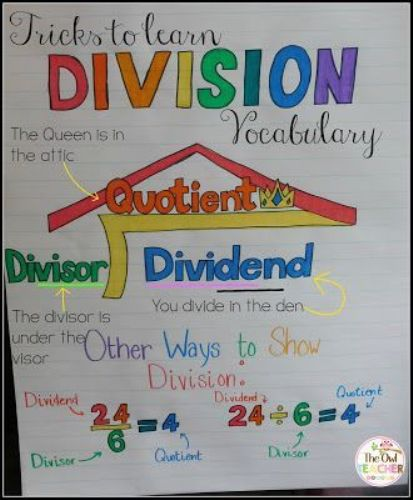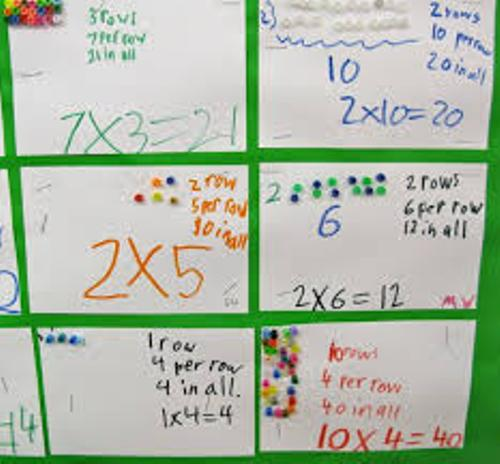Friday, September 23rd 2016. | Mathematics

Facts about Division tell the readers about the one of the four basic operations in arithmetic. Besides division, people have to learn multiplication, subtraction and addition. If you have 20 apples and you want to give them to four groups, each group will get five apples.  Some people define division as the process for fraction evaluation. Here are other interesting facts about division:

## Facts about Division 1: multiplication and division relationship

Multiplication and division are related each other because the inverse of division is multiplication.

### Facts about Division 2: an undefined division

The division of natural number is undefined if it is divided by zero. However, the division can be defined in some contexts.division learning

## Facts about Division 3: the dividend, divisor and quotient

The quotient will be gained when the divisor divides the dividends in division. If 20: 5 = 4, so the quotient is four, the divisor is five and the dividend is 20.

### Facts about Division 4: the remainder

The division is not always complete. In some cases, the remainder occurs when the divisor is not fully contained in the dividend. Get facts about dice here.division

## Facts about Division 5: the application of division

The application of division is not limited for dividing apples. You can also use it to divide abstract and physical objects.

### Facts about Division 6: the abstract contexts

The vector spaces and fields are included as the abstract contexts where division can be applied. Other contexts include the complex and real numbers. Check facts about decimals here.## Facts about Division 7: educating students

It is not easy to teach division to the students because it has different process from multiplication, subtraction and addition. The concept of fraction should be introduced to the students since a remainder may be gained from division. In science and algebra, the division is presented by having the dividend over the divisor with the fraction bar or horizontal line in between.

### Facts about Division 8: tools for calculating division

You can calculate division by using abacus. If you want divide two numbers, it is okay to use logarithm tables.division pictures

## Facts about Division 9: faster division

If you want to enjoy faster calculation of division, use the computer. It only takes seconds to do a long division.

### Facts about Division 10: the fast division instruction

You have to make sure that the computer is fitted with fast division instruction if you want to get faster division calculation.division pic